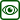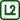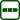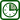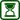## Magdeburg hemispheres

### Experiment number : 4304

• #### Goal of experiment

The goal of the experiment is to show how the difference in gas pressure (in this case, the lower pressure inside the hemispheres) manifests itself.

• #### Theory

In 1654, Otto von Guericke, mayor of Magdeburg, carried out the following experiment. He put together two copper hemispheres with a diameter of 51 cm and pumped air out of the space between them. He then hitched four pairs of horses to each hemisphere and showed that these 16 horses were unable to pull the hemispheres apart. This experiment proved the existence of the Earth’s atmosphere. The copper hemispheres were held together by the pressure of the surrounding air (atmospheric pressure).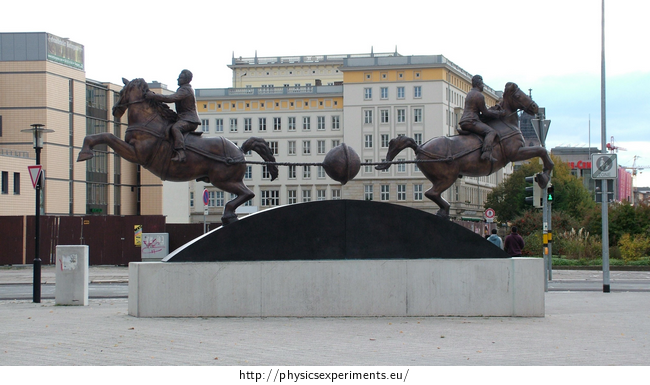A similar experiment can be done with two suction cups for carrying glass. However, this experiment differs from the original experiment with hollow copper hemispheres in design. While the air from inside the actual Magdeburg hemispheres has been pumped out, the amount of air between the suction cups does not change. We just enlarge the cavity between the suction cups, which reduces the air pressure inside.

In both of these experiments the air pressure difference is important. If the pressure between the two hemispheres (or suction cups) is lower than the pressure in their surroundings, the outside air will push on the hemispheres with a compressive force that is directly proportional to the pressure difference between the outside and inside. The hemispheres of cross-sectional area A can only be pulled apart if this compressive force is exceeded.

• #### Tools

• Two suction cups for the transport of glass panels

• Two students who will try to separate suction cups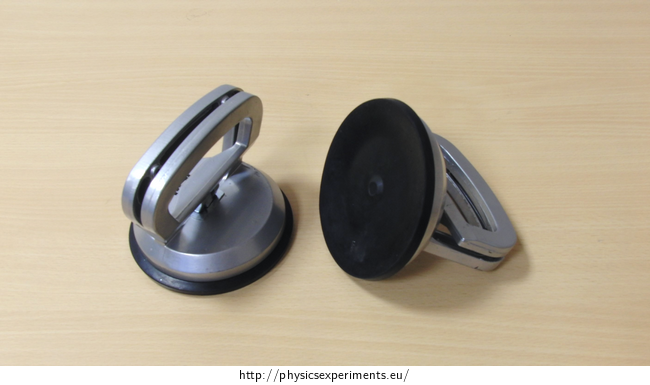• #### Procedure

1. First clean the rubber surface of the suction cups.

2. Put the suction cups together with the rubber surface and suck the air out.

3. Each of the suction cups is grabbed by one student. They both try to separate the suction cups from each other.

• #### Example of results

Students are unlikely to be able to pull the suction cups apart. If they do, it is possible that they were not held together tightly enough before being engaged.

• #### Calculation of the force required to pull the hemispheres apart

We calculate approximately what the air pressure is between our suction cups.

If we know the magnitude of the force F, that could separate the suction cups from each other, we can find the difference in pressure outside and inside the suction cups Δp. The force F is equal to the product of the pressure difference Δp and the area A over which this pressure acts:

$F\,=\,\mathrm{\Delta}pA.\tag{1}$

By pressure difference we mean the difference between the atmospheric pressure pa and the pressure between the suction cups ps. The relation (1) can therefore be written in the form

$F\,=\,(p_{\mathrm{a}}-p_{\mathrm{s}})A.\tag{2}$

From equation (2) we express the pressure between the suction cups ps:

$p_{\mathrm{s}}\,=\,p_{\mathrm{a}}-\frac{F}{A}.\tag{3}$

The producer of the glass suction cups we used for this experiment states that they have a carrying capacity of 50 kg. Therefore, suppose that if we apply a pulling force greater than F ≈ 490 N to the suction cups, we can separate them.

We calculate the area A of one suction cup A as the area of a circle whose radius r is in our case 6 cm:

$A = πr^2.$

Substitute into relation (3) and calculate the numerical values

$p_{\mathrm{s}}\,=\,p_{\mathrm{a}}-\frac{F}{{\pi}r^2}\,\dot{=}\,\left(101325-\frac{490}{\pi{\cdot}0.06^2}\right)\,\mathrm{Pa}\,\dot{=}\,58\,\mathrm{kPa}.$

We have calculated that the air pressure between the suction cups is approximately 58 kPa, which is slightly more than half of the atmospheric pressure. However, the suction cups probably have a higher carrying capacity than 50 kg; the producer certainly provides this value with a margin. The air pressure between the suction cups is then probably less than our calculated value.

• #### Technical notes

Students should be careful while pulling the suction cups. They might be able to separate the suction cups and then they might fall down. It’s necessary to ensure that there are no furniture edges and students don’t hurt themselves.